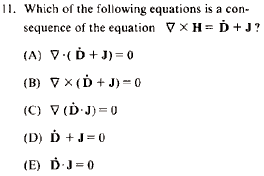GR 8677927796770177 | # Login | Register

GR8677 #11
Problem
 GREPhysics.NET Official Solution Alternate SolutionsElectromagnetism}Vector Calculus

There are two identities from vector calculus one has to know by heart. The one directly applicable to this problem is:

Plug in the equation given in the problem to the identity above to get 0.

(The other identity, not quite as useful for this problem, but perhaps useful for subsequent problems, is: )Alternate Solutions
 There are no Alternate Solutions for this problem. Be the first to post one!
ernest21
2019-08-10 03:09:22
So we take the fact that we know the frequency has to increase from above. Now we look at the speed. i\'m using tilt controls
 slegeza2019-08-26 20:07:25 Why are you spamming sketchy links on every comment you leave on this site?fredluis
2019-08-08 12:36:37
I\'m surprised no one noticed this one... As x -> infinite, acceration must approach g (a -> g), so choice is the only possibility. tree trimmingjoshuaprice153
2019-08-08 06:23:04
I will must share this blog and the information i found here really has no value in money but more than it. Thanks for this nice effort which you put here in the shape of this post. security guard Orlandonakib
2012-09-29 12:56:20
I solved it using Maxwell's equations. The given equation is Ampere's law in differential form. You can see the electric current term J in there. This current term is enabled by the fact that there are electric monopoles. Monopoles are allowed to exist if . Only (A) has that property. If you can remember this, this method is at least as quick as using the vector identity mentioned by Yosun.pam d
2011-09-23 19:46:58
Just to be clear for people that might be foggy on vector calc, the answer is (A) for reasons already explained.casanovo
2008-10-29 14:52:17
The identity didn't show up, so here it is in words:

The divergence of the curl is always zero.Furious
2007-10-30 22:58:19
Left out an = 0 in that last part.So we take the fact that we know the frequency has to increase from above. Now we look at the speed. i\'m using tilt controlsLaTeX syntax supported through dollar sign wrappers $, ex.,$\alpha^2_0$produces . type this... to get...$\int_0^\infty\partial\Rightarrow\ddot{x},\dot{x}\sqrt{z}\langle my \rangle\left( abacadabra \right)_{me}\vec{E}\frac{a}{b}\$